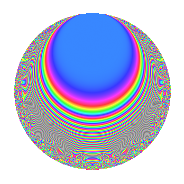# Properties

 Label 403.2.h.aLevel 403 Weight 2 Character orbit 403.h Analytic conductor 3.218 Analytic rank 0 Dimension 30 CM No

# Related objects

## Newspace parameters

 Level: $$N$$ = $$403 = 13 \cdot 31$$ Weight: $$k$$ = $$2$$ Character orbit: $$[\chi]$$ = 403.h (of order $$3$$ and degree $$2$$)

## Newform invariants

 Self dual: No Analytic conductor: $$3.21797120146$$ Analytic rank: $$0$$ Dimension: $$30$$ Relative dimension: $$15$$ over $$\Q(\zeta_{3})$$ Sato-Tate group: $\mathrm{SU}(2)[C_{3}]$

## $q$-expansion

The dimension is sufficiently large that we do not compute an algebraic $$q$$-expansion, but we have computed the trace expansion.

 $$\operatorname{Tr}(f)(q) =$$ $$30q - 6q^{2} - 2q^{3} + 22q^{4} + 7q^{5} + 6q^{7} - 24q^{8} - 13q^{9} + O(q^{10})$$ $$\operatorname{Tr}(f)(q) =$$ $$30q - 6q^{2} - 2q^{3} + 22q^{4} + 7q^{5} + 6q^{7} - 24q^{8} - 13q^{9} - 3q^{10} - 3q^{11} + 12q^{12} + 15q^{13} + 3q^{14} + 4q^{15} + 22q^{16} + 4q^{17} - 6q^{18} + 5q^{19} + 10q^{20} - 15q^{21} + 2q^{22} - 6q^{23} - 6q^{24} - 20q^{25} - 3q^{26} + 4q^{27} + 13q^{28} + 26q^{29} + 28q^{30} + 19q^{31} - 74q^{32} + 2q^{33} - 21q^{34} - 30q^{35} + 37q^{36} - 4q^{37} - 4q^{38} - 4q^{39} - 17q^{40} + 7q^{41} + 44q^{42} + 15q^{43} - 70q^{44} + 15q^{45} - 58q^{46} - 24q^{47} + 31q^{48} - 29q^{49} + 2q^{50} + 25q^{51} + 11q^{52} + 4q^{53} + 34q^{54} + 13q^{55} - 41q^{56} + 8q^{57} - 46q^{58} + 27q^{59} + 78q^{60} + 8q^{61} + 20q^{62} - 112q^{63} + 60q^{64} - 7q^{65} + 60q^{66} + 29q^{67} + 41q^{68} - 23q^{69} - 112q^{70} + 29q^{71} + 14q^{72} - 10q^{73} + 25q^{74} - 18q^{75} + 24q^{76} - 72q^{77} + 9q^{79} - 11q^{81} - 38q^{82} - 13q^{83} - 62q^{84} + 108q^{85} - 38q^{86} - 18q^{87} + 32q^{88} + 6q^{89} + 7q^{90} + 12q^{91} + 2q^{92} + 37q^{93} - 154q^{94} - 28q^{95} - 105q^{96} - 84q^{97} + 50q^{98} + 10q^{99} + O(q^{100})$$

## Embeddings

For each embedding $$\iota_m$$ of the coefficient field, the values $$\iota_m(a_n)$$ are shown below.

For more information on an embedded modular form you can click on its label.

Label $$a_{2}$$ $$a_{3}$$ $$a_{4}$$ $$a_{5}$$ $$a_{6}$$ $$a_{7}$$ $$a_{8}$$ $$a_{9}$$ $$a_{10}$$
118.1 −2.75405 1.04794 + 1.81508i 5.58479 1.03612 1.79462i −2.88606 4.99881i 2.05805 + 3.56464i −9.87267 −0.696336 + 1.20609i −2.85353 + 4.94246i
118.2 −2.54177 −0.358627 0.621161i 4.46058 −1.54812 + 2.68142i 0.911547 + 1.57885i −1.09448 1.89569i −6.25421 1.24277 2.15255i 3.93495 6.81554i
118.3 −2.19969 −0.332345 0.575638i 2.83864 2.12692 3.68393i 0.731057 + 1.26623i −0.0876538 0.151821i −1.84476 1.27909 2.21546i −4.67856 + 8.10350i
118.4 −1.54088 −0.945700 1.63800i 0.374297 0.246770 0.427419i 1.45721 + 2.52395i −2.41271 4.17893i 2.50501 −0.288697 + 0.500038i −0.380242 + 0.658599i
118.5 −1.20534 −1.24518 2.15671i −0.547152 0.667125 1.15549i 1.50087 + 2.59957i 1.94340 + 3.36606i 3.07019 −1.60094 + 2.77291i −0.804114 + 1.39277i
118.6 −1.11604 0.521580 + 0.903404i −0.754455 −0.0866186 + 0.150028i −0.582104 1.00823i −0.0906774 0.157058i 3.07408 0.955908 1.65568i 0.0966698 0.167437i
118.7 −0.899619 1.46679 + 2.54055i −1.19069 −1.03352 + 1.79011i −1.31955 2.28553i 1.98012 + 3.42966i 2.87040 −2.80293 + 4.85482i 0.929773 1.61041i
118.8 0.0439466 0.820034 + 1.42034i −1.99807 0.865000 1.49822i 0.0360377 + 0.0624192i −2.17574 3.76849i −0.175702 0.155089 0.268621i 0.0380138 0.0658419i
118.9 0.165462 −0.350644 0.607334i −1.97262 −1.35983 + 2.35530i −0.0580182 0.100491i 0.231883 + 0.401634i −0.657317 1.25410 2.17216i −0.225001 + 0.389713i
118.10 0.352077 −1.65894 2.87336i −1.87604 1.09161 1.89072i −0.584073 1.01164i −1.14275 1.97930i −1.36466 −4.00415 + 6.93538i 0.384330 0.665679i
118.11 1.21548 −0.671133 1.16244i −0.522608 1.76937 3.06463i −0.815749 1.41292i 0.724247 + 1.25443i −3.06618 0.599162 1.03778i 2.15063 3.72500i
118.12 1.41262 −1.31623 2.27977i −0.00449076 −1.72422 + 2.98644i −1.85934 3.22047i 2.33584 + 4.04580i −2.83159 −1.96492 + 3.40333i −2.43568 + 4.21871i
118.13 1.58004 1.43561 + 2.48655i 0.496524 1.71834 2.97625i 2.26832 + 3.92885i 0.908007 + 1.57271i −2.37555 −2.62196 + 4.54137i 2.71504 4.70259i
118.14 2.10012 0.700181 + 1.21275i 2.41051 −0.938510 + 1.62555i 1.47047 + 2.54692i 0.810515 + 1.40385i 0.862110 0.519492 0.899787i −1.97098 + 3.41385i
118.15 2.38763 −0.113336 0.196303i 3.70079 0.669573 1.15973i −0.270604 0.468700i −0.988047 1.71135i 4.06087 1.47431 2.55358i 1.59869 2.76902i
222.1 −2.75405 1.04794 1.81508i 5.58479 1.03612 + 1.79462i −2.88606 + 4.99881i 2.05805 3.56464i −9.87267 −0.696336 1.20609i −2.85353 4.94246i
222.2 −2.54177 −0.358627 + 0.621161i 4.46058 −1.54812 2.68142i 0.911547 1.57885i −1.09448 + 1.89569i −6.25421 1.24277 + 2.15255i 3.93495 + 6.81554i
222.3 −2.19969 −0.332345 + 0.575638i 2.83864 2.12692 + 3.68393i 0.731057 1.26623i −0.0876538 + 0.151821i −1.84476 1.27909 + 2.21546i −4.67856 8.10350i
222.4 −1.54088 −0.945700 + 1.63800i 0.374297 0.246770 + 0.427419i 1.45721 2.52395i −2.41271 + 4.17893i 2.50501 −0.288697 0.500038i −0.380242 0.658599i
222.5 −1.20534 −1.24518 + 2.15671i −0.547152 0.667125 + 1.15549i 1.50087 2.59957i 1.94340 3.36606i 3.07019 −1.60094 2.77291i −0.804114 1.39277i
See all 30 embeddings
 $$n$$: e.g. 2-40 or 990-1000 Embeddings: e.g. 1-3 or 222.15 Significant digits: Format: Complex embeddings Normalized embeddings Satake parameters Satake angles

## Inner twists

This newform does not have CM; other inner twists have not been computed.

## Hecke kernels

This newform can be constructed as the kernel of the linear operator $$T_{2}^{15} + \cdots$$ acting on $$S_{2}^{\mathrm{new}}(403, [\chi])$$.